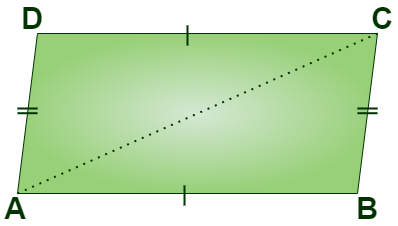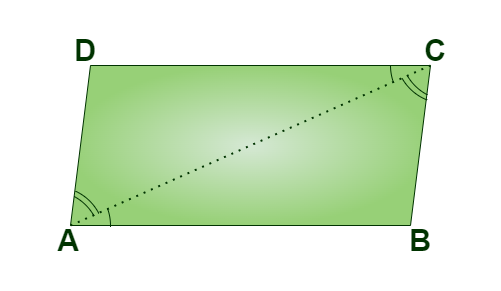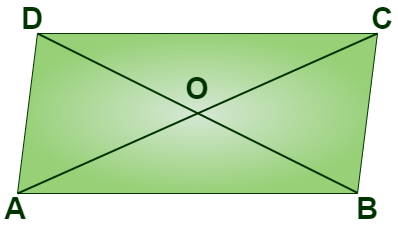GeeksforGeeks App
Open AppBrowser
Continue

## Related Articles

Parallelogram is a  quadrilateral in which opposite sides are parallel and congruent and the opposite angles are equal. A parallelogram is formed by the intersection of two pairs of parallel lines.

## Important Properties of a Parallelogram

A parallelogram is a quadrilateral in which opposite sides are parallel and equal in length. Also, opposite angles are also equal. Few of the important properties of a parallelogram are:

• Opposite sides of a parallelogram are equal in length and are parallel to each other.
• Opposite angles in a parallelogram are equal.
• Sum of all interior angles of a parallelogram is 360°.
• Consecutive angles of a parallelogram are supplementary (180°).

## Properties of Parallelogram Diagonals

Various properties of the diagonal of a parallelogram are:

• Both diagonals of a parallelogram bisect each other.
• The parallelogram is bisected into two congruent triangles by each diagonal.
• By parallelogram law, Sum of squares of the diagonal of a parallelogram is equal to the sum of squares of all the sides of a parallelogram.

## Theorems on Properties of a Parallelogram

Few important theorems on properties of a Parallelogram are discussed below in this article:

### Theorem 1: Opposite sides of a parallelogram are equal.

Given: ABCD is a parallelogram

To Prove: AB = CD & DA = BCProof:

Given ABCD is a parallelogram. Therefore,

AB || DC  &  AD || BC

Now,  AD || BC and AC is intersecting A and C respectively.

∠DAC = ∠BCA…(i)                  [Alternate Interior Angles]

Now, AB || DC and AC is intersecting A and C respectively.

∠BAC = ∠D …(ii)                    [Alternate Interior Angles]

∠DAC = ∠BCA [ From (i) ]
AC = AC [ Common Side ]
∠DCA = ∠BAC [ From (ii) ]

So, by ASA(Angle-Side-Angle) criterion of congruence

AB = CD & DA = BC [ Corresponding part of congruent triangles are equal ]

Hence Proved

### Converse of Theorem 1: If opposite sides are equal in a quadrilateral, then it is a parallelogram

Given: Opposite sides in a quadrilateral ABCD are equal, AB = CD, and BC = AD.

To Prove: Quadrilateral ABCD is a parallelogram.

In quadrilateral ABCD, AB = CD and AD = BC. In triangles ABC, and CDA we have

AC = AC (Common sides)
AB = CD (since alternate interior angles are equal)

So by the SSS congruency criterion, triangles ABC, and CDA are congruent, thus by CPCT corresponding angles of triangles are equal. Thus, ∠BAC = ∠DCA, and ∠BCA = ∠DAC.

Now AB || CD, BC || AD and thus ABCD is a parallelogram.

### Theorem 2: Opposite angles of a parallelogram are equal.Given: ABCD is a parallelogram

To Prove:  ∠A = ∠C  and ∠B = ∠D

Proof:

Given ABCD is a parallelogram. Therefore,

AB || DC  &  AD || BC

Now, AB || DC and AD is Intersecting them at A and D respectively.

∠A + ∠D = 180º               …(i)             [ Sum of consecutive interior angles is 180º]

Now, AD || BC and DC is Intersecting them at D and C respectively.

∠D + ∠C = 180º              …(ii)            [ Sum of consecutive interior angles is 180º]

From (i) and (ii) , we get

∠A + ∠D = ∠D  +  ∠C

So,  ∠A = ∠C

Similarly, ∠B = ∠D

∠A = ∠C and  ∠B = ∠D

Hence Proved

### Converse of Theorem 2: If the opposite angles in a quadrilateral are equal, then it is a parallelogram

Given: In the quadrilateral ABCD ∠A = ∠C and ∠B = ∠D

To Prove: ABCD is a parallelogram.

Proof:

Given ∠A = ∠C and ∠B = ∠D in quadrilateral ABCD. We have to prove ABCD is a parallelogram

∠A + ∠B + ∠C + ∠D = 360º (given ∠A = ∠C and ∠B = ∠D )

2(∠A + ∠B) =360º

∠A + ∠B = 180º.

Thus AD || BC. Similarly, we can show that AB || CD.

Hence, AD || BC, and AB || CD. Therefore ABCD is a parallelogram.

### Theorem 3: Diagonals of a parallelogram bisect each other.Given: ABCD is a parallelogram

To Prove: OA = OC & OB = OD

Proof:

AB || DC  &  AD || BC

Now, AB || DC and AC is intersecting A and C respectively.

∠BAC = ∠DCA                               [ Alternate Interior Angles are equal ]

So, ∠BAO = ∠DCO

Now,  AB || DC and BD is intersecting B and D respectively.

∠ABD = ∠CDB                               [ Alternate Interior Angles are equal ]

So, ∠ABO = ∠CDO

Now, in  ΔAOB &  ΔCOD we have,

∠BAO = ∠DCO                               [ Opposite sides of a parallelogram are equal ]

AB = CD

∠ABO = ∠CDO

So, by ASA(Angle-Side-Angle) congruence criterion

ΔAOB  ΔCOD

OA = OC and OB = OD

Hence Proved

### Converse of Theorem 3: If the diagonals of a quadrilateral bisect each other, then it is a parallelogram.

Given: The diagonals AC and BD bisect each other.

To Prove: ABCD is a parallelogram.

Proof:

If the diagonals AB and CD bisect each other. Then in Δ AOB, and Δ COD

AO = CO (Diagonals bisect each other)
BO = DO (Diagonals bisect each other)
∠AOB =∠COD (vertically opposite angles)

Thus, by SAS congruency criterion, triangles are congruent. So  ∠CAB = ∠DCA, and ∠DBA = ∠CDB. Hence, AB || CD, and BC || AD. Thus ABCD is a parallelogram.

## Solved Example on Properties of Parallelogram

Example 1: ABCD is a quadrilateral with AB = 10 cm. Diagonals of ABCD bisect each other at right angles. Then find the perimeter of ABCD.

Solution:

We know that, if diagonals of a quadrilateral bisect each other at right angles then it is a rhombus.

Thus, ABCD is a rhombus and  AB = BC = CD = DA.

Thus, the perimeter of ABCD = 4(AB) = 4(10) = 40 cm

Example 2: Find area of a parallelogram where the base is 6 cm and the height is 12 cm.

Solution:

Given, Base = 6 cm and Height = 12 cm.

We know,

Area = Base x Height

Area = 6  × 12

Area = 72 cm2

## FAQs on Properties of a Parallelogram

Question 1: What is a parallelogram?

A parallelogram is a quadrilateral that has in which opposite sides are parallel and equal. And opposite angles in a parallelogram equal. It is also considered as a cyclic quadrilateral.

Question 2: Can a Rhombus be called a Parallelogram?

Opposite sides of a rhombus are equal and parallel, and its opposite angles are also equal. So it is considered a parallelogram.

Question 3: What are the four important properties of a parallelogram?

The four important properties of the parallelogram are:

• Opposite sides of a parallelogram are parallel and congruent
• Consecutive angles of a parallelogram add up to 180 degrees
• Opposite angles of a parallelogram are equal
• Diagonals of a parallelogram bisect each other.

Question 4: What is the order of rotational symmetry in a parallelogram?

Order of rotational symmetry is a parallelogram is 2.

Question 5: Does a parallelogram have reflectional symmetry?

No, reflectional symmetry is not possible in a parallelogram.

Question 6: Are the Diagonals of a Parallelogram Equal?